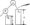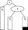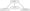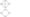# Data Structures and Algorithms Feb 2011

Unit-I

1.  a) Determine the frequency counts for all statements in the following program segments.

i)    For (z =1 ;/<=»;i++)

for (j = 1 ;j < = i ;j ++)

for (k = l ;k< — j; k ++)

x = x + 1 ;

ii)   i= 1;

while (/ < = n)

{

x = x+ 1;

i = i+ 1;

}

Also compute the time complexity for both the segments.

b)  Justify whether the statements below are correct

i)   3n + 2 = 0 (n)

ii)  3n+2 = Ω(n)

iii)  3n+2 =Ɵ(«)

iv)  10n2+4n+2 – 0(n)2

v) 6×2n+-n2 = Ω(2n)

vi)  6×2n+n2≠0(n2)

OR

a) Design a suitable array representation for storing polynomials of the form P(x) = anxn +aixi + awhere n≥ 1000, I<<n and a 0 is a constant. Draw schematic diagrams.

b)  Use the representation in (a) above and write an algorithm to add two such polynomials. Find its time complexity and space complexity

c)   Write an algorithm to compute the frequency of occurrence of ASCII characters in a given string. No space shall be used for the characters not present in the input string and the list shall contain the frequencies in the order of ASCII value of characters in the input stream.

Unit-II

2. a) Design a suitable: array representation to store

i) Symmetric matrix

ii) Lower triangular matrix.

b)  Write algorithms for the following cases :

ii) Adding a symmetric matrix to a non – symmetric matrix.

iii) Adding a symmetric matrix to a lower triangular matrix.

OR

a )How a sparse matrix can be represented using a linked structure? Explain with Suitable schematic diagrams.

b) Write an algorithm to transpose a sparse matrix represented as a linked structure ^^the resulting matrix shall be ordered on the row value if the input is ordered on row Value.

c) Derive the expression for computing the address of A [i1,] [i2]…………….. [in] in an n-dimensional array A [u1] [u2]…………….. [un]. Assume that the first element in the array is A    .

Unit-III

3. a) i) Design a representation for storing 2 stacks in a single array.

(3) j ii) Develop conditions for overflow for the representation.

iii) Develop algorithm for insertion into the designated stack.

b) i) What advantages are offered by circular queues over linear queues? What are 1 imitations of circular queues?

ii) Develop algorithm for insertion into and deletion from a circular queue.

OR

A programming language does not accept parenthesis in the expressions. However, it has a facility of outputting fully parenthesized expressions of the input expressions. The programmer can then check the correctness of the input expression.

Develop the algorithm for outputting fully parenthesized expressions from expressions without parenthesis. Please ensure that the precedence rules are not violated. Show the correctness of your algorithm taking 2 examples.

Unit – IV

4. a) Assume an array of size n. Show all the steps in storing the following sequence in the array to be used a binary tree:

71,27, 23, 29, 180, 143, 78,28,30.

Assume n is larger than the number of elements. Compute the location of each element.

b)  Write algorithm for insertion of an element into a binary tree represented in an array.

c)   Write algorithm for inorder, postorder and pre – order traversal of a binary tree stored in an array. Test your results with the binary tree constructed in (a) above.

OR

5. a) Draw the height balanced tree for the following trees:

i)Where AR = Right subtree of A, height = h

BL = Left subtree of B, height =h+1

BR = Right subtree of B, height = h.

ii)AL = Left subtree of A; height = h

BL = Left subtree of B ; height = h

BR = Right Subtree of B; height = h+1

New node is shown shaded. Write the procedural steps,

b)    Define a B-Tree. What is the significance of the order of B tree.

sert the keys 62,5,85,75 one at a time into the following B-tree of order 5.Unit-V

5. a) Represent following graph usingb)  Use any representation in (a) and write algorithm to perform depth first search on the graph.

c)   Assume that A is the starting node, find the result of depth first search on the graph using the algorithm developed in (b).

OR

a)  Write short notes on the applications of the following sorting algorithms.

i) Heap Sort.

ii) Quick Sort

iii) Merge Sort

iv) Selection Sort.

b)  Compare the time and space complexities of the following sorting algorithms: Bubble sort, insertion sort, merge sort, selection sort, shell sort, Quick sort. Heap sort.